# NCERT Solution - Accounting Ratios Notes | Study Accountancy Class 12 - Commerce

## Commerce: NCERT Solution - Accounting Ratios Notes | Study Accountancy Class 12 - Commerce

The document NCERT Solution - Accounting Ratios Notes | Study Accountancy Class 12 - Commerce is a part of the Commerce Course Accountancy Class 12.
All you need of Commerce at this link: Commerce

Page No.: 238
Long Questions:
Question 1: What do you mean by Ratio Analysis?
Answer: Ratio Analysis is a technique of financial analysis. It describes the relationship between various items of Balance Sheet and Income Statements. It helps us in ascertaining profitability, operational efficiency, solvency, etc. of a firm. It may be expressed as a fraction, proportion, percentage and in times. It enables budgetary controls by assessing qualitative relationship among different financial variables. Ratio Analysis provides vital information to various accounting users regarding the financial position and viability and performance of a firm. It also lays down the basic framework for decision making and policy designing by management.

Question 2: What are the various types of ratios?
Answer: Accounting ratios are classified in the following two ways.
II. Functional Classification
I. Traditional Classification: This classification is based on the financial statements, i.e. Profit and Loss Account and Balance Sheet. The Traditional Classification further bifurcates accounting ratios on the basis of the accounts to which the elements of a ratio belong. On the basis of accounts of financial statements, the Traditional Classification bifurcate accounting ratios as:
a. Income Statement Ratios: These are those ratios whose all the elements belong only to the Trading and Profit and Loss Account, like Gross Profit Ratio, etc.
b. Balance Sheet Ratios: These are those ratios whose all the elements belong only to the Balance Sheet, like Current Ratio, Debt Equity Ratio, etc.
c. Composite Ratios: These are those ratios whose elements belong both to the Trading and Profit and Loss Account as well as to the Balance Sheet, like Debtors Turnover Ratio, etc.
II. Functional Classification: This classification reflects the functional need and the purpose of calculating ratio. The basic rationale to compute ratio is to ascertain liquidity, solvency, financial performance and profitability of a business. Consequently, the Functional Classification classifies various accounting ratios as:
a. Liquidity Ratio: These ratios are calculated to determine short term solvency.
b. Solvency Ratio: These ratios are calculated to determine long term solvency.
c. Activity Ratio: These ratios are calculated for measuring the operational efficiency and efficacy of the operations. These ratios relate to sales or cost of goods sold.
d. Profitability Ratio: These ratios are calculated to assess the financial performance and the financial viability of the business.

Question 3: What relationships will be established to study:
a. Inventory Turnover
b. Debtor Turnover
c. Payables Turnover
d. Working Capital Turnover.

Answer: a. Inventory Turnover Ratio: This ratio is computed to determine the efficiency with which the stock is used. This ratio is based on the relationship between cost of goods sold and average stock kept during the year.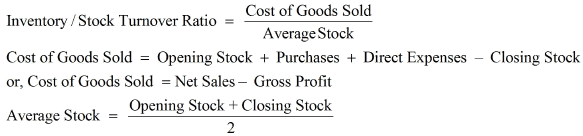b. Debtors Turnover Ratio or Trade Receivable Turnover RatioThis ratio is computed to determine the rate at which the amount is collected from the debtors. It establishes the relationship between net credit sales and average accounts receivables.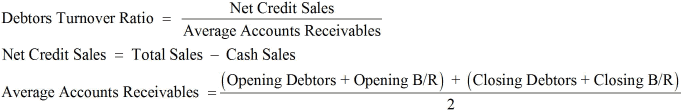c. Trade Payables Turnover Ratio: This ratio is known as Creditors Turnover Ratio. It is computed to determine the rate at which the amount is paid to the creditors. It establishes the relationship between net credit purchases and average accounts payables.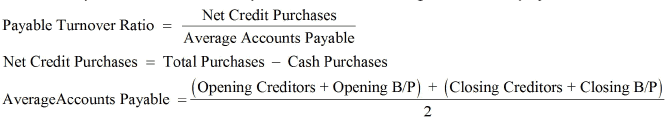d. Working Capital Turnover Ratio: This ratio is computed to determine how efficiently the working capital is utilised in making sales. It establishes the relationship between net sales and working capital.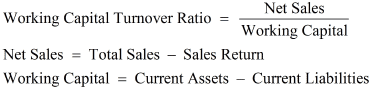Question 4: The liquidity of a business firm is measured by its ability to satisfy its long-term obligations as they become due. What are the ratios used for this purpose?
Answer: The liquidity of a business firm is measured by its ability to pay its long term obligations. The long term obligations include payments of principal amount on the due date and payments of interests on the regular basis. Long term solvency of any business can be calculated on the basis of the following ratios.
a. Debt-Equity Ratio- It depicts the relationship between the borrowed fund and owner's funds. The lower the debt-equity ratio higher will be the degree of security to the lenders. A low debt-equity ratio implies that the company can easily meet its long term obligations.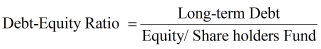b. Total Assets to Debt Ratio- It shows the relationship between the total assets and the long term loans. A high Total Assets to Debt Ratio implies that more assets are financed by the owner's fund and the company can easily meet its long-term obligations. Thus, a higher ratio implies more security to the lenders.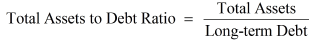c. Interest Coverage Ratio- This ratio depicts the relationship between amount of profit utilised for paying interest and amount of interest payable. A high Interest Coverage Ratio implies that the company can easily meet all its interest obligations out of its profit.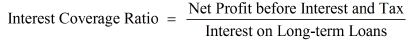Question 5: The average age of inventory is viewed as the average length of time inventory is held by the firm or as the average number of day's sales in inventory. Why?
Answer: Inventory Turnover Ratio: This ratio is computed to determine the efficiency with which the stock is used. This ratio is based on the relationship between cost of goods sold and average stock kept during the year.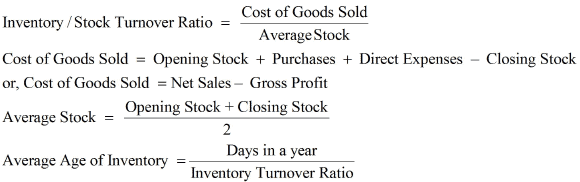It shows the rate with which the stock is turned into sales or the number of times the stock in turned into sales during the year. In other words, this ratio reveals the average length of time for which the inventory is held by the firm.

Page No.: 238
Long Questions:
Question 1: What are liquidity ratios? Discuss the importance of current and liquid ratio.
Answer: Liquidity ratios are calculated to determine the short-term solvency of a business, i.e. the ability of the business to pay back its current dues. Liquidity means easy conversion of assets into cash without any significant loss and delay.
Short-term creditors are interested in ascertaining liquidity ratios for timely payment of their debts.
Liquidity ratio includes
1. Current Ratio
2. Liquid Ratio or Quick Ratio
1. Current Ratio- It explains the relationship between current assets and current liabilities. It is calculated as: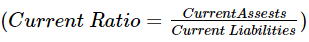Currents Assets are those assets that can be easily converted into cash within a short period of time like, cash in hand, cash at bank, marketable securities, debtors, stock, bills receivables, prepaid expenses. etc.
Current Liabilities are those liabilities that are to be repaid within a year like, bank overdraft, bills payables, Short-term creditors, provision for tax, outstanding expenses etc.
Importance of Current Ratio
It helps in assessing the firm's ability to meet its current liabilities on time. The excess of current assets over current liabilities provide a sense of safety and security to the creditors. The ideal ratio of current assets over current liabilities is 2:1. It means that the firm has sufficient funds to meet its current liabilities. A higher ratio indicates poor investment policies of management and low ratio indicates shortage of working capital and lack of liquidity.
2. Liquid Ratio- It explains the relationship between liquid assets and current liabilities. It indicates whether a firm has sufficient funds to pay its current liabilities immediately. It is calculated as: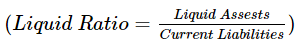Liquid Assets = Current Assests - Stock - Prepaid Expenses
Importance of Liquid Ratio
It helps in determining whether a firm has sufficient funds if it has to pay all its current liabilities immediately.
It does not include stock, since it takes comparatively more time to convert the stock into cash. Further prepaid expenses are also not included in liquid assets, since these cannot be converted into cash. The ideal Liquidity Ratio is considered to be 1:1. It means that the firm has a rupee in form of liquid assets for every rupee of current liabilities.
Question 2: How would you study the solvency position of the firm?
Answer: Solvency position of a firm is studied with the help of the Solvency Ratios. Solvency ratios are the measures of the long-term financial position of the firm in terms of its ability to pay its long-term liabilities. In other words, the solvency of the firm is measured by its ability to pay its long term obligation on the due date. The long term obligations include payments of principal amount on the due date and payments of interests on the regular basis. Long term solvency of any business can be calculated on the basis of the following ratios.
a. Debt-Equity Ratio- It depicts the relationship between the borrowed fund and owner's funds. The lower the debt-equity ratio higher will be the degree of security to the lenders. A low debt-equity ratio implies that the company can easily meet its long term obligations.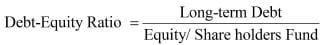Equity or the Shareholders Fund includes Preference Share Capital, Equity Share Capital, Capital Reserve, Securities Premium, General Reserve less Accumulated Loss and Fictitious Assets.
b. Total Assets to Debt Ratio- It shows the relationship between the total assets and the long term loans. A high Total Assets to Debt Ratio implies that more assets are financed by the owner's fund and the company can easily meet its long-term obligations. Thus, a higher ratio implies more security to the lenders.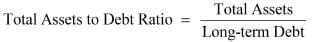Total Assets includes all fixed and current assets except fictitious assets like, Preliminary Expenses, Underwriting Commission, etc.
Debt includes all long-term loans that are to be repaid after one year. It includes debentures, mortgage loans, bank loans, loans from other financial institutions, etc.
c. Interest Coverage Ratio- This ratio depicts the relationship between amount of profit utilise for paying interest and amount of interest payable. A high Interest Coverage Ratio implies that the company can easily meet all its interest obligations out of its profit.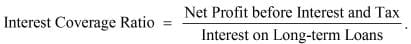d. Proprietary Ratio- It shows the relationship between the Shareholders Fund and the Total Assets. This ratio reveals the financial position of a business. The higher the ratio the higher will be the degree of safety for the creditors. It is calculated as: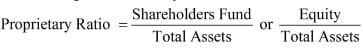Total Assets includes all fixed and current assets except fictitious assets like, Preliminary Expenses, Underwriting Commission, etc.

Question 3: What are important profitability ratios? How are they worked out?
Answer: Profitability ratios are calculated on the basis of profit earned by a business. This ratio gives a percentage measure to assess the financial viability, profitability and operational efficiency of the business. The various important Profitability Ratios are as follows:
1. Gross Profit Ratio
2. Operating Ratio
3. Operating Profit Ratio
4. Net Profit Ratio
5. Return on Investment or Capital Employed
6. Earnings per Share Ratio
7. Dividend Payout Ratio
8. Price Earnings Ratio
1. Gross Profit Ratio- It shows the relationship between Gross Profit and Net Sales. It depicts the trading efficiency of a business. A higher Gross Profit Ratio implies a better position of a business, whereas a low Gross Profit Ratio implies an inefficient unfavourable sales policy.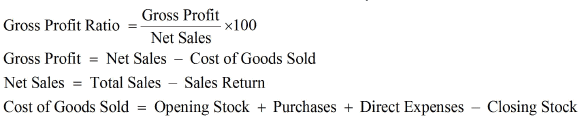2. Operating Ratio- It shows the relationship between Cost of Operation and Net Sales. This ratio depicts the operational efficiency of a business. A low Operating Ratio implies higher operational efficiency of the business. A low Operating Ratio is considered better for the business as it enables the business to be left with a greater amount after covering its operation costs to pay for interests and dividends.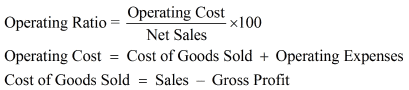3. Operating Profit Ratio- It shows the relationship between the Operating Profit and Net Sales. It helps in assessing the operational efficiency and the performance of the business.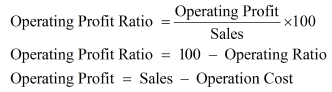4. Net Profit Ratio- It shows the relationship between net profit and sales. Higher ratio is better for firm. It depicts the overall efficiency of a business and acts as an important tool to the investors for analysing and measuring the viability and performance of the business.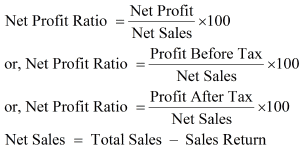5. Return on Investment or Capital Employed- It shows the relationship between the profit earned and the capital employed to earn that profit. It is calculated as: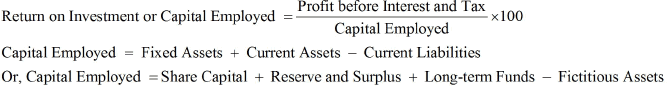This ratio depicts the efficiency with which the business has utilised the capital invested by the investors. It is an important yardstick to assess the profit earning capacity of the business.
6. Earning per Shares- It shows the relationship between the amount of profit available to distribute as dividend among the equity shareholders and number of equity shares.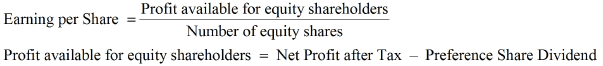7. Dividend Payout Ratio- It shows the relationship between the dividend per share and earnings per share. This ratio depicts the amount of earnings that is distributed in the form of dividend among the shareholders. A high Dividend Payout Ratio implies a better position and goodwill of the business for the shareholders.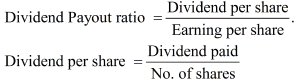8. Price Earning Ratio- It shows the relationship between the market price of a share and the earnings per share. This ratio is the most common tool that is used in the stock markets. This ratio depicts the degree of reliance and trust that the shareholders have on the business. This ratio reflects the expectation of the shareholders regarding the rise in the future prices of the company's shares. A higher Price Earning Ratio definitely enables a company to enjoy favourable position in the market.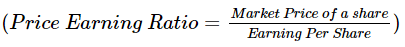Question 4: The current ratio provides a better measure of overall liquidity only when a firm's inventory cannot easily be converted into cash. If inventory is liquid, the quick ratio is a preferred measure of overall liquidity. Explain.
Answer: Current Ratio- It explains the relationship between current assets and current liabilities. It is calculated as: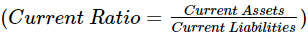Currents Assets are those assets that are easily converted into cash within a short period of time like cash in hand, cash at bank, marketable securities, debtors, stock, bills receivables, prepaid expenses. etc.
Current Liabilities are those liabilities that are to be repaid within a year like bank overdraft, bills payables, Short-term creditors, provision for tax, outstanding expenses etc.
Liquid Ratio- It explains the relationship between liquid assets and current liabilities. It indicates whether a firm has sufficient funds to pay its current liabilities immediately. It is calculated as: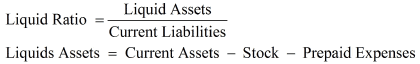Generally, Current Ratio is preferable for such type of business where the stock or the inventories cannot easily be converted into cash like heavy machinery manufacturing companies, locomotive companies, etc. This is because, the heavy stocks like machinery, heavy tools etc. cannot be easily sold off. But on the other hand, the businesses where the stock can be easily realised or sold off regard Liquid Ratio to be more suitable measure to reveal their liquidity position. For example, the inventories of a service sector company is very liquid as there are no stock kept for sale, so they prefer Liquid Ratio as a measure of overall liquidity.
Moreover, sometimes companies prefer to resort to Liquid Ratio instead of Current Ratio, if the prices of the stock held are prone to fluctuate. This is because if the prices of the inventories fluctuate more, then this may affect their liquidity position of the business and may reduce (or overcast) the Current Ratio. Consequently, they prefer Liquid Ratio as it excludes inventories and stocks.
Thirdly, if the stock forms the major portion of a company's current assets, then they would prefer Current Ratio and not Liquid Ratio. This is because their current assets mostly consist of stock. The Liquid Ratio of such company will be very low as liquid assets exclude stock. This will reduce their Liquid Ratio and may create a bad image for the creditors. In such a case, Current Ratio provides better measure of overall liquidity.

Page No: 238
Numerical Questions:
Question 1: Following is the Balance Sheet of Raj Oil Mills Limited as at March 31, 2016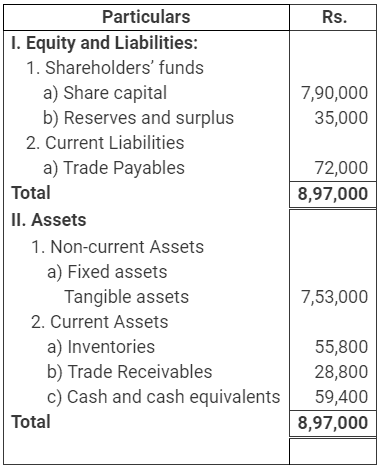Calculate Current Ratio.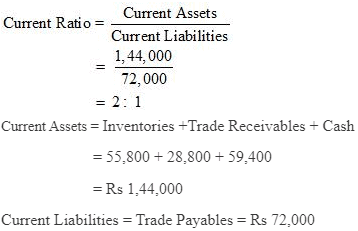Page No: 239
Numerical Questions:
Question 2: Following is the Balance Sheet of Title Machine Ltd. as at March 31, 2017.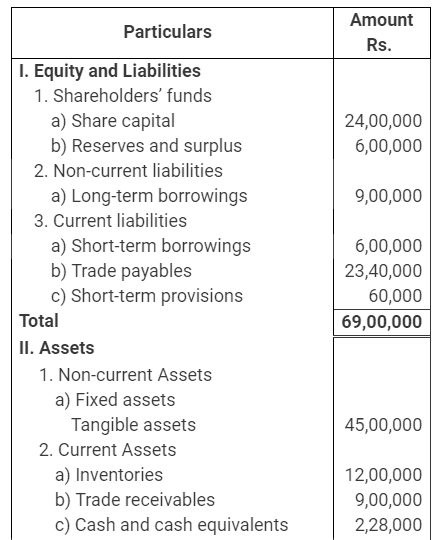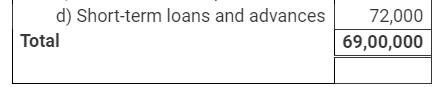Calculate Current Ratio and Liquid Ratio.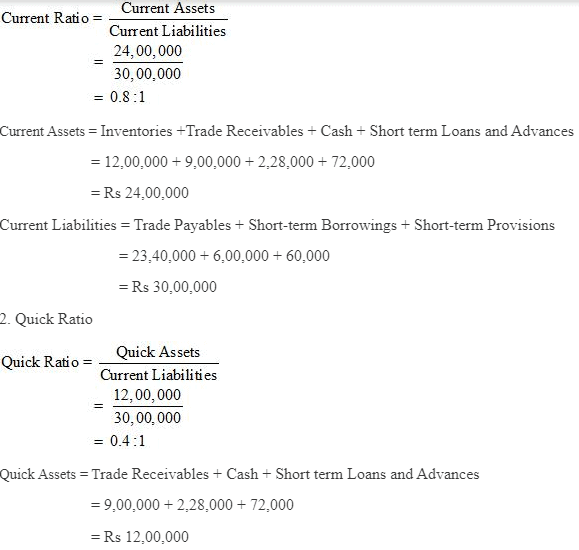Page No.: 239

Numericals Questions:
Question 1: Current Ratio is 3.5:1 Working Capital is Rs 9,00,000. Calculate the amount of Current Assets and Current Liabilities.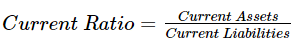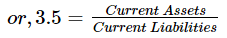or, Current Assets = 3.5 Current Liabilities (1)
Working Capital = Current Assets - Current Liabilities
Working Capital = 90,000
or, Current Assets - Current Liabilities = 90,000
or, 3.5 Current Liabilities - Current Liabilities = 90,000 (from 1)
or, 2.5 Current Liabilities = 90,000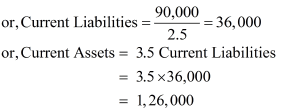Question 2: Shine Limited has a current ratio 4.5:1 and quick ratio 3:1; if the stock is 36,000, calculate current liabilities and current assets.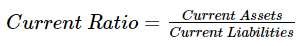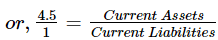or, 4.5 Current Liabilities = Current Assets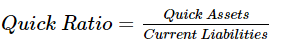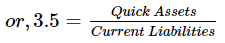or, 3 Current Liabilities = Quick Assets
Quick Assets = Current Assets- Inventory  = Current Assets - 36,000
Current Assets − Quick Assets = 36,000
or, 4.5 Current Liabilities − 3 Current Liabilities = 36,000
or, 1.5 Current Liabilities = 36,000
or, Current Liabilities = 24,000
Current Assets = 4.5 Current Liabilities
or, Current Assets = 4.5 x 24,000
= 1,08,000

Note: The solution given in the book is incorrect as it from the given figures Current Assets is ascertained to be Rs 1,08,000 and Current Liabilities Rs 24,000.

Question 3: Current liabilities of a company are Rs 75,000. If current ratio is 4:1 and liquid ratio is 1:1, calculate value of current assets, liquid assets and inventory
Answer: Current Ratio = Current Assets /Current Liabilities
or, 4 = Current Assets / 75,000
or, 4 × 75,000 = Current Assets
or, Current Assets = 3,00,000
Liquid Ratio = Liquid Assets / Current Liabilities
or, 1 = Liquid Assets / 75,000
Liquid Assets = 75,000
Inventory = Current Assets − Liquid Assets
= 3,00,000 − 75,000
= 2,25,000

Page No.: 240
Numericals Questions:

Question 1: Handa Ltd.has stock of Rs 20,000. Total liquid assets are Rs 1,00,000 and quick ratio is 2:1. Calculate current ratio.
Answer: Quick Ratio = Quick Assets / Current Liabilities
or, 2 =  1,00,000 / Current Liabilities
or, Current Liabilities =  1,00,000 / 2
= 50,000
Current Assets = Liquid Assets + Inventory
= 1,00,000 + 20,000
= 1,20,000
Current Ratio = Current Assets / Current Liabilities
=1,20,000/ 50,000
=2.4/1= 2.4:1

Question 2: Calculate debt equity ratio from the following information:

 Rs Total Assets 15,00,000 Current Liabilities 6,00,000 Total Debts 12,00,000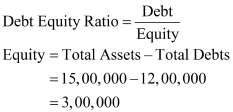Long Term Depts = Total Depts - Current Liabilities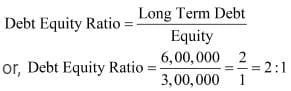Question 3: Calculate Current Ratio if:
Inventory is Rs 6,00,000; Liquid Assets Rs 24,00,000; Quick Ratio 2:1.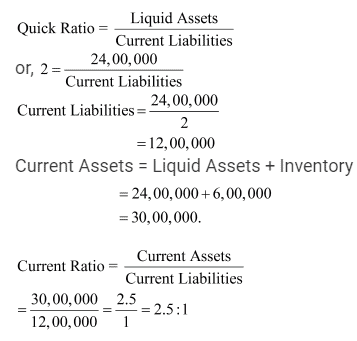Question 4: Compute Stock Turnover Ratio from the following information: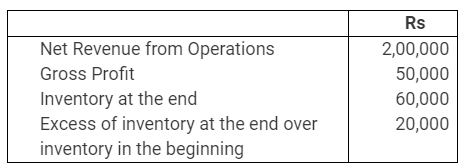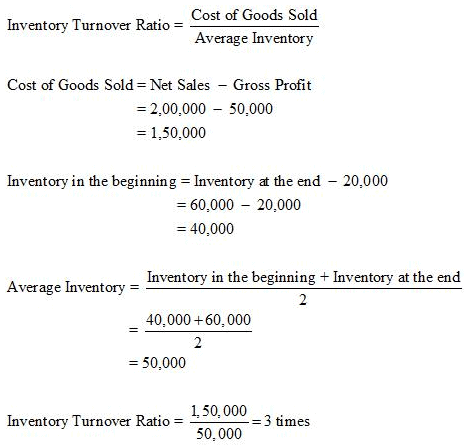Question 5: Calculate following ratios from the following information:
(i) Current ratio (ii) Acid test ratio (iii) Operating Ratio (iv) Gross Profit Ratio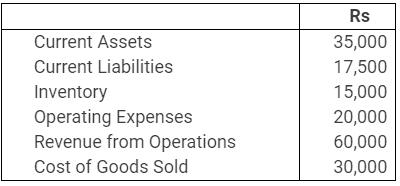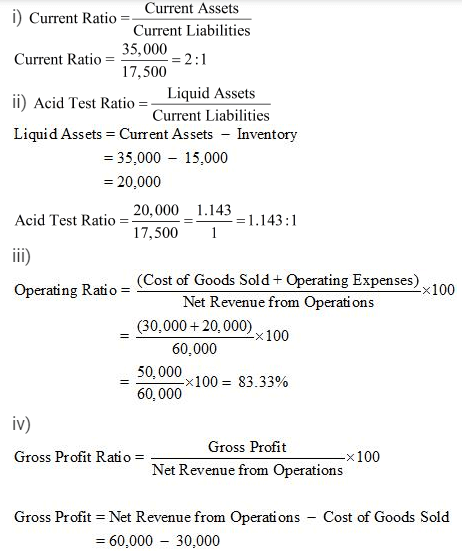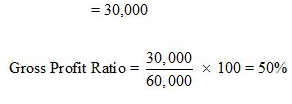Question 6: From the following information calculate:
(i) Gross Profit Ratio (ii) Inventory Turnover Ratio (iii) Current Ratio (iv) Liquid Ratio (v) Net Profit Ratio (vi) Working capital Ratio: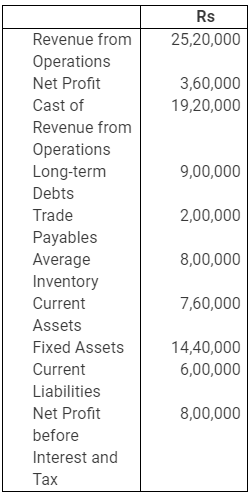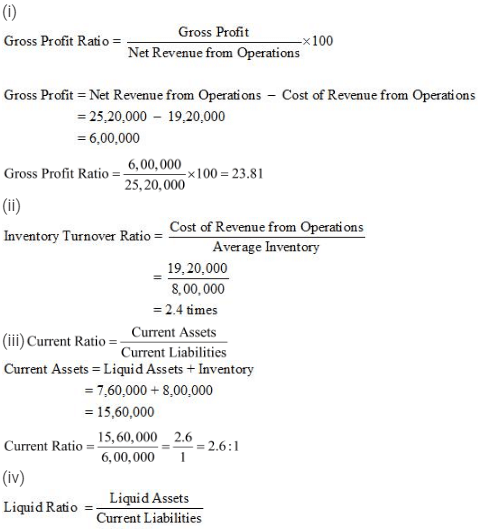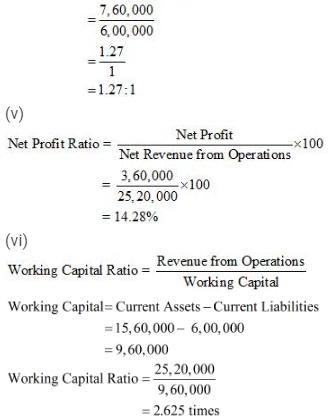NoteThere is a misprint in the question given in the textbook. The figure of Rs '760,000' represents the value of 'Liquid Assets' and not 'Current Assets'. The above solution has been worked out accordingly and the answer given as per the textbook is same as per the above solution.

Page No.: 241
Numericals Questions:
Question 1: Compute Gross Profit Ratio, Working Capital Turnover Ratio, Debt Equity Ratio and Proprietary Ratio from the following information: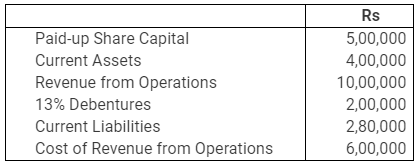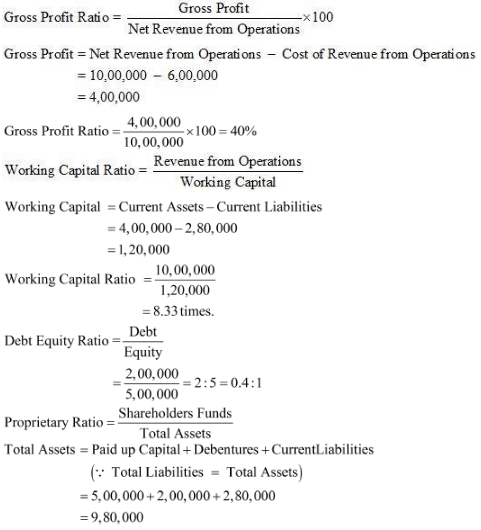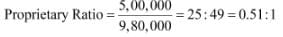Question 2: Calculate Inventory Turnover Ratio if:
Inventory in the beginning is Rs 76,250, Inventory at the end is 98,500, Gross Revenue from Operations is Rs 5,20,000, Return Inwards is Rs 20,000, Purchases is Rs 3,22,250.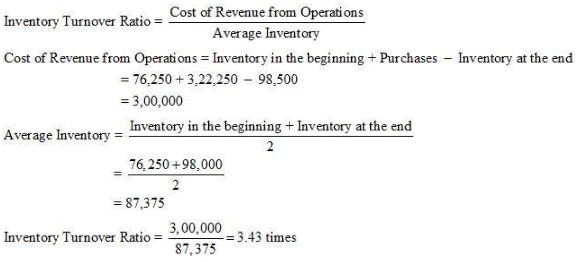Page No. : 241
Numerical Questions :
Question 1 : Calculate Inventory Turnover Ratio from the data given below: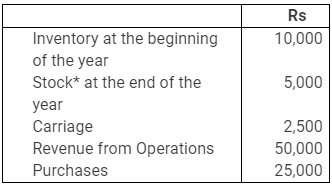*Since the very first item is Inventory in the beginning, so this item should be Inventory at the end.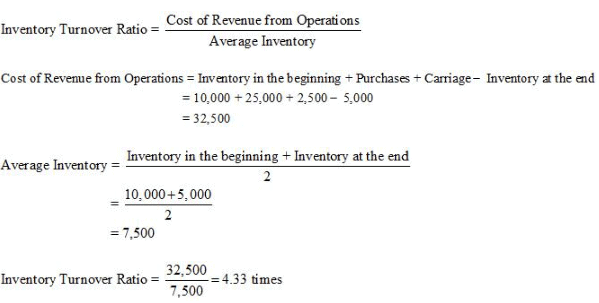Question 2 : A trading firm’s average inventory is Rs 20,000 (cost). If the inventory turnover ratio is 8 times and the firm sells goods at a profit of 20% on sale, ascertain the profit of the firm.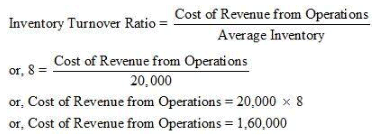Let Sale Price be Rs 100
Then Profit is Rs 20
Hence, the Cost of Revenue from Operations = Rs 100 − Rs 20 = Rs 80
If the Cost of Revenue from Operations is Rs 80, then Revenue from Operations = 100
If the Cost of Revenue from Operations is Rs 1, then Revenue from Operations = 100/80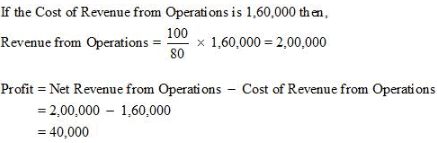Question 3 : You are able to collect the following information about a company for two years: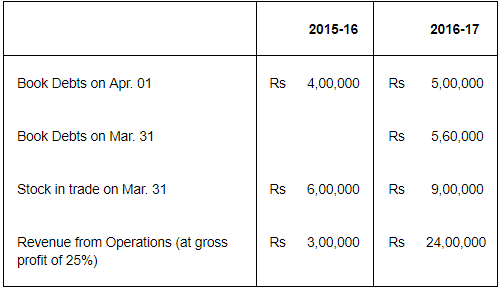Calculate Inventory Turnover Ratio and Trade Receivables Turnover Ratio if in the year 2015-16 stock in trade increased by Rs 2,00,000.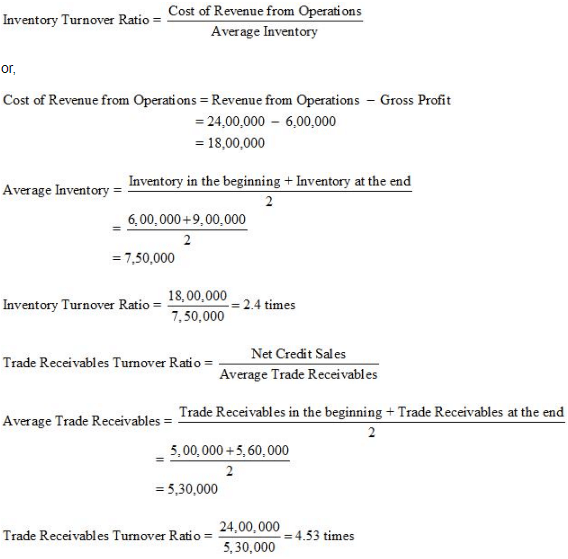Note: It has been assumed that all sales are credit sales
Page no. 242
Numericals Questions
Question 1 : The following Balance Sheet and other information, calculate following ratios:
(i) Debt-Equity Ratio
(ii) Working Capital Turnover Ratio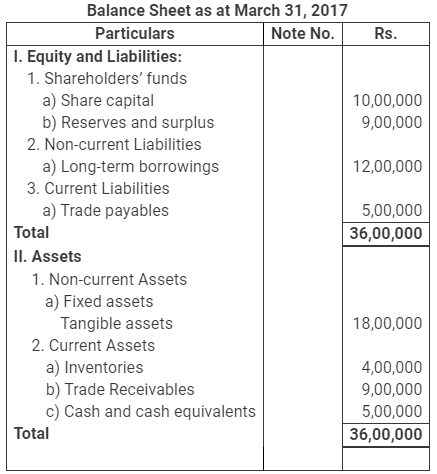Additional Information: Revenue from Operations Rs. 18,00,000 Calculate:
i) Debt-Equity Ratio
ii) Working Capital Turnover Ratio
(Debt-Equity Ratio 0.63:1; Working Capital Turnover Ratio 1.39 times; Trade
Receivables Turnover Ratio 2 times)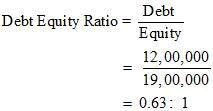Debt = Long Term Borrowings = Rs 12,00,0000
Equity = Share Capital + Reserve and Surplus
= 10,00,000 + 9,00,000
= Rs 19,00,000
2. Working Capital Turnover Ratio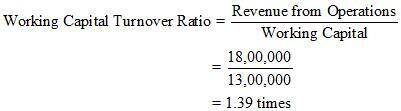Revenue from Operations = Rs 18, 00,000
Working Capital = Current Assets – Current Liabilities
= 18,00,000 – 5,00,000
= Rs 13,00,000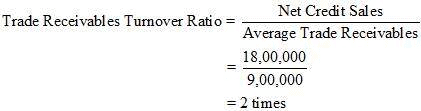Net Credit Sales = Rs 18,00,000
Average Trade Receivables = Rs 9,00,000
Notes:
1. Revenue from Operations are assumed to be revenue generated from credit sales.

2. The amount of trade receivables given in the Balance Sheet is assumed to be Average Trade Receivables.

Question 2 : From the following information, calculate the following ratios:
i) Quick Ratio
ii) Inventory Turnover Ratio
iii) Return on Investment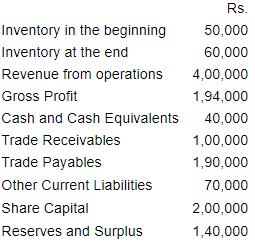(Balance in the Statement of Profit & Loss A/c)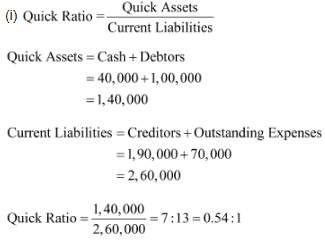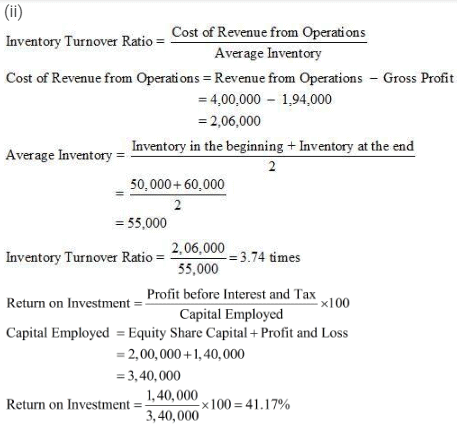Page no. 243
Numerical Questions :
Question 3 : From the following, calculate
(a) Debt Equity Ratio (b) Total Assets to Debt Ratio (c) Proprietary Ratio.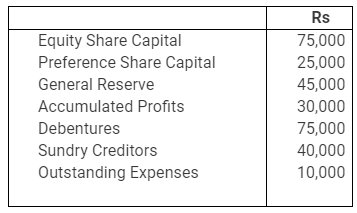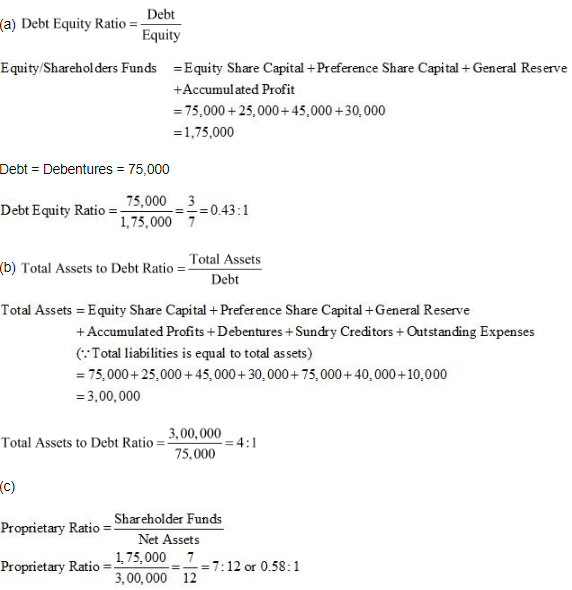Question 4 : Cost of Revenue from Operations is Rs 1,50,000. Operating expenses are Rs 60,000. Revenue from Operations is Rs 2,50,000. Calculate Operating Ratio.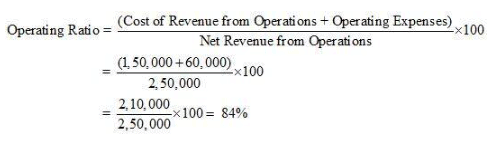Question 5 : The following is the summerised transactions and Statement of Profit and Loss Account for the year ending March 31, 2007 and the Balance Sheet as on the basis of following information, calculate:
(i) Gross Profit Ratio (ii) Current Ratio (iii) Acid Test Ratio (iv) Inventory Turnover Ratio (v) Fixed Assets Turnover Ratio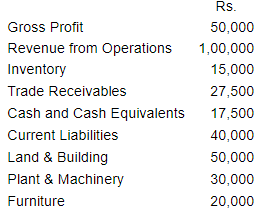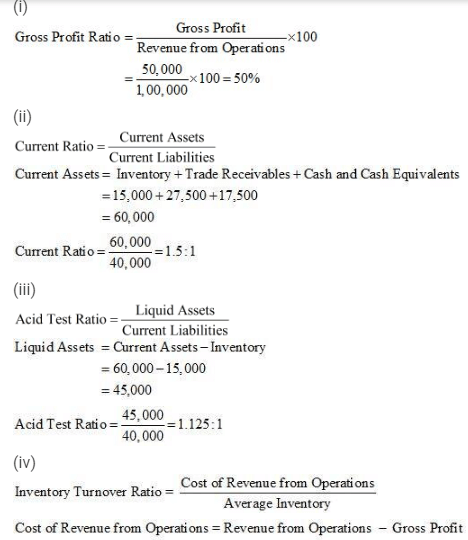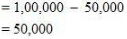Average Inventory = 15,000*
*Note: Since values for inventory in the beginning and inventory at the end is not given, the amount of inventory is assumed to be average inventory.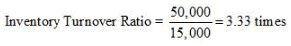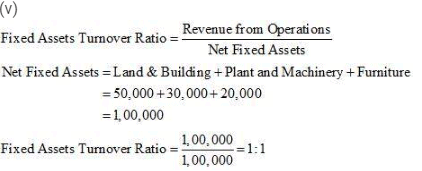Question 6 : From the following information calculate Gross Profit Ratio, Inventory Turnover Ratio and Trade Receivables Turnover Ratio.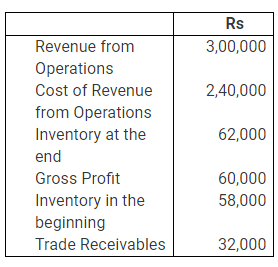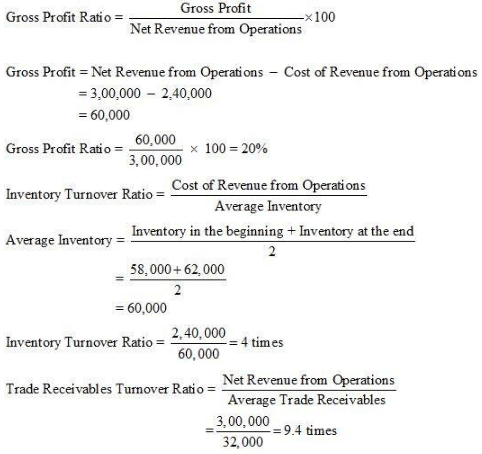Note: In the solution, Trade Receivables are assumed as the Average Trade Receivables.

The document NCERT Solution - Accounting Ratios Notes | Study Accountancy Class 12 - Commerce is a part of the Commerce Course Accountancy Class 12.
All you need of Commerce at this link: CommerceUse Code STAYHOME200 and get INR 200 additional OFF

## Accountancy Class 12

62 videos|81 docs|68 tests

Track your progress, build streaks, highlight & save important lessons and more!

,

,

,

,

,

,

,

,

,

,

,

,

,

,

,

,

,

,

,

,

,

;